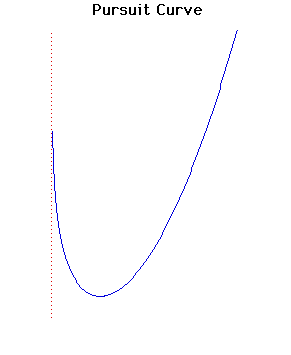# Curves

### Pursuit CurveCartesian equation:
$y = cx^{2} - \log(x)$

### Description

If $A$ moves along a known curve then $P$ describes a pursuit curve if $P$ is always directed towards $A$ and $A$ and $P$ move with uniform velocities. These were considered in general by the French scientist Pierre Bouguer in 1732.

The case here is where $A$ is on a straight line and was studied by Arthur Bernhart.

Pierre Bouguer was a French scientist who was the first to attempt to measure the density of the Earth using the deflection of a plumb line due to the attraction of a mountain. He made measurements in Peru in 1740. A more successful use of this method by the astronomer Maskelyne placed the density between 4.5 and 5.

### Associated Curves

Definitions of the Associated curves